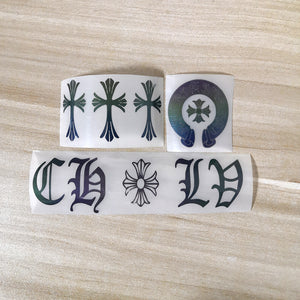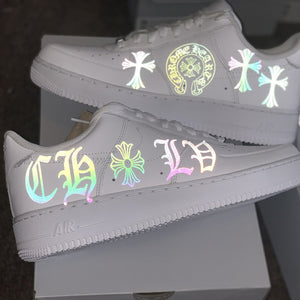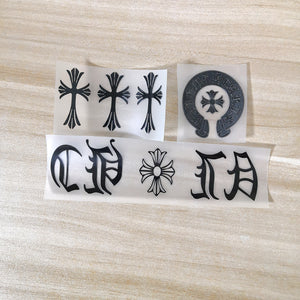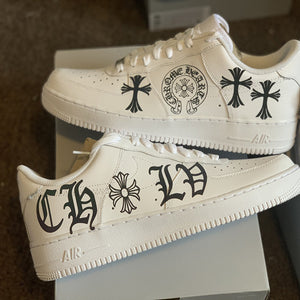" .... Rainbow Reflective Chrome Hearts Logo Iron On Patches for DIY / Custom – theshoesgirl
Order Over \$120 can cover free FedEx shipping!
Order Over \$120 can cover free FedEx shipping!# Rainbow Reflective Chrome Hearts Logo Iron On Patches for DIY / Custom Air Force 1 Chrome Hearts Them

Regular price \$15.00 \$12.00 Save \$3.00

ONLY 199892 LEFT

 Hours   Minutes   Seconds

Sale Ends Once The Timer Hits Zero!

ONLY 199892 LEFT

Get these easy use iron on colorful rainbow reflective Chrome Hearts patches to DIY to create your own shoes. Best size for DIY or custom Air Force 1 & Vans.

<<<<<<<<<<<<<<<<<<<<<<<<<<<<<<<<<<<<<<<<<<<<<<<<<<<<<<<<

### Why use these Chrome Hearts patches:

1. Perfect sizes for sneakers: AF1, Vans, Converse or other shoes.

2. Also can be used on cloth, socks. hoodies, jeans, tshirt....

3. Durable, waterproof and flexible.

4. Easy use, just need to iron on top. No need any transfer paper, iron on directly when you received them.

5. Get these colorful reflective dragon patches to DIY, save lot's money to buy dragon shoes.

6. Beautiful: reflective different colors with different angles.

<<<<<<<<<<<<<<<<<<<<<<<<<<<<<<<<<<<<<<<<<<<<<<<<<<<<<<<<

The set includes total 9 symbols

The shoes are not included in the set.

<<<<<<<<<<<<<<<<<<<<<<<<<<<<<<<<<<<<<<<<<<<<<<<<<<<<<<<

<<<<<<<<<<<<<<<<<<<<<<<<<<<<<<<<<<<<<<<<<<<<<<<<<<<<<<<

### How to use:

1. Position the dragon patches on the shoes with tape

2. Iron on the top of the dragon, please keep temperature 140-150℃

3. When the ironed places are totally cool down, then to peel the vinyl, the dragon patches will stick on the shoes.

4. If use normal iron, please turn off the steam, keep the temperature above, use the tip and be careful and patient, for it's heavy to control, better use the mini iron.

<<<<<<<<<<<<<<<<<<<<<<<<<<<<<<<<<<<<<<<<<<<<<<<<<<<<<<<

### Free shipping

people are currently looking at this product

Estimated delivery time 7-15 days

Guaranteed Safe Checkout